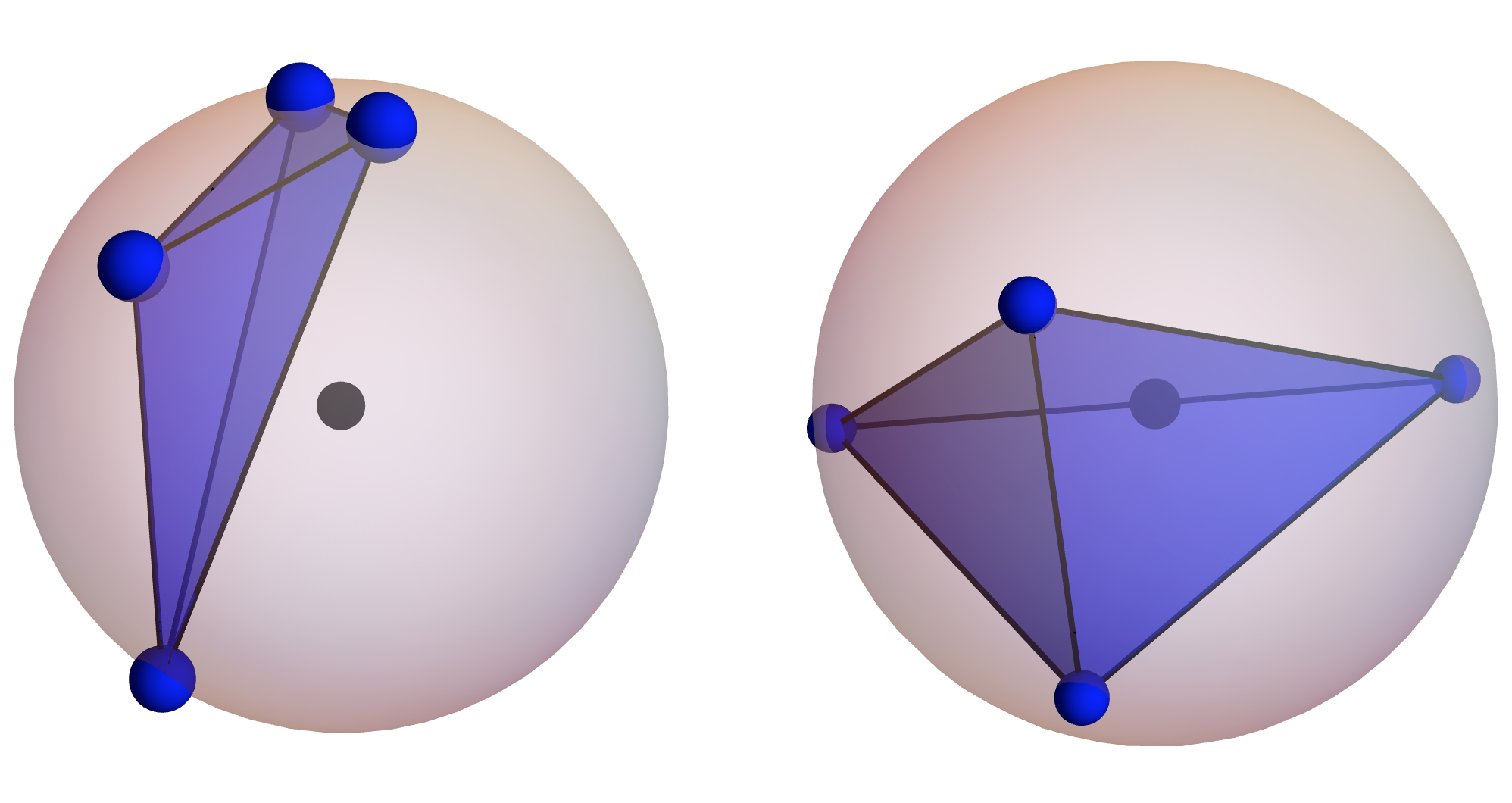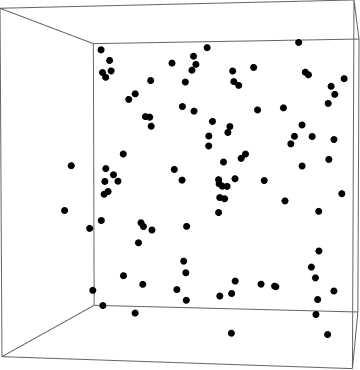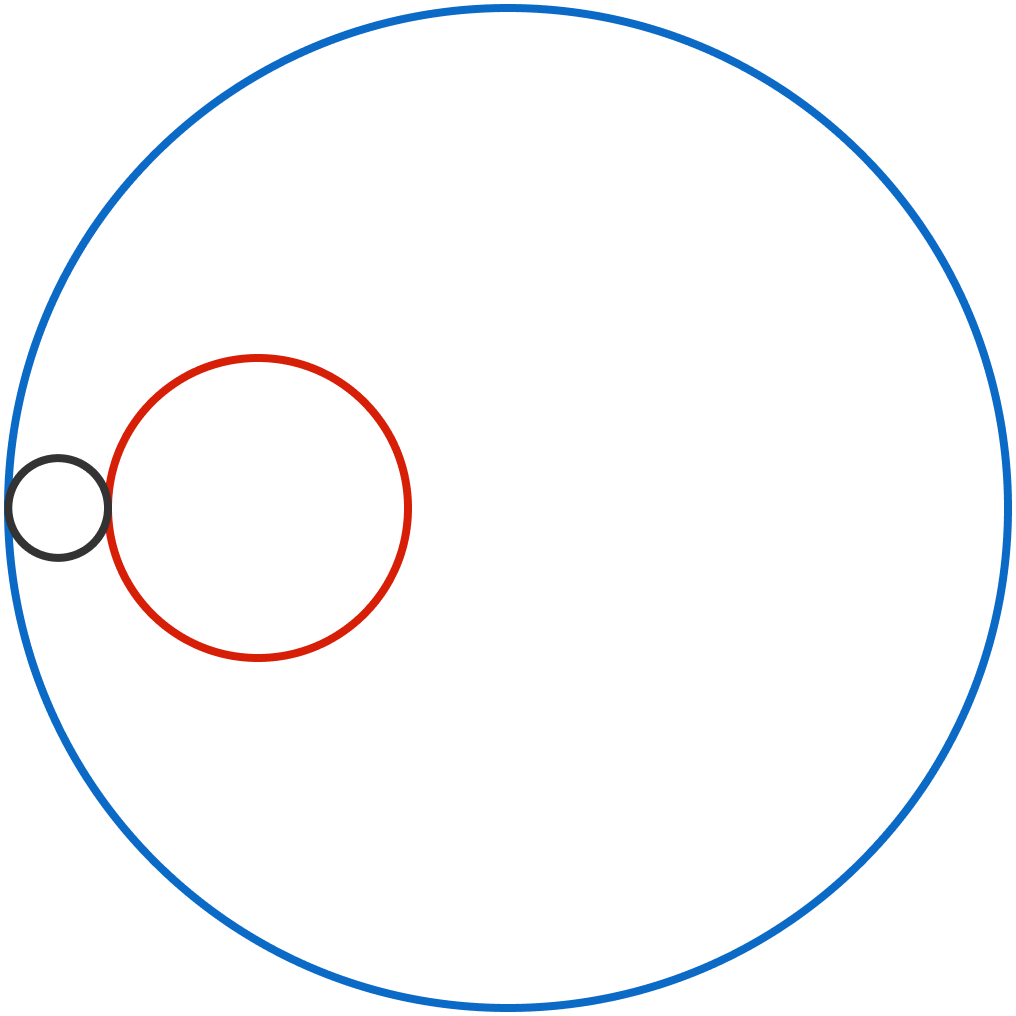# Problems of the Week

Contribute a problem

4 points are randomly chosen on a sphere to form a tetrahedron.

What is the probability the center of the sphere will be in the tetrahedron?On the left, the center of the sphere isn't inside the random tetrahedron. On the right, it is.

The well-known equation of state for an ideal gas, $PV = Nk_BT,$ is only true on average. In reality, the pressure fluctuates from moment to moment as more or fewer gas particles hit the wall in a given instant.Suppose we measure the pressure on the walls of the container at $m$ different times $\mathcal{P} = \{P_{t_1}, P_{t_2},\ldots, P_{t_m}\}.$ We can measure the magnitude of the pressure fluctuations by finding the standard deviation $\sigma_P = \sqrt{\langle P^2\rangle - \langle P\rangle^2}.$

How will the size of the fluctuations shrink relative to the mean as we increase the number of gas particles?

In other words, if $N$ denotes the number of particles, what is $\dfrac{\sigma_P}{\langle P\rangle}$ proportional to?

A particularly long integer ends with an 8. Shifting this 8 digit to the leftmost position creates a new number which is twice the original. Find the smallest possible value of the original number.

Clarification: This 8-shift procedure looks as follows: $123{\color{#D61F06}8} \longrightarrow {\color{#D61F06}8}123.$In the figure, the radii of the red circle and the blue circle are 3 and 10, respectively, and the distance between their centers is 5.

The black circle is externally tangent to the red circle and internally tangent to the blue circle. The path traced by the center of the black circles is a closed curve whose area is $X\pi.$

What is $X?$

Let $a_1,a_2,...,a_n$ be distinct positive integers such that $a_1+a_2+\cdots+a_n=2018.$

Find the maximum value of $a_1\times a_2\times \cdots \times a_n.$

If this is equal to $\frac{a!}{b}$, where $a$ and $b$ are distinct positive integers and $a+b$ is minimized, write your answer as $\frac{ab}{2}.$

×